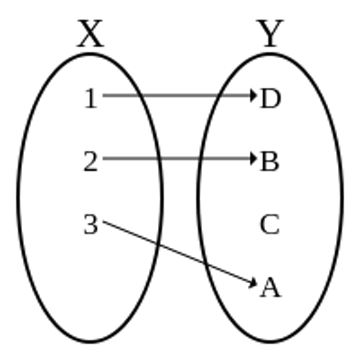# Computable Injective FunctionLet $f$ be a one-to-one (Injective) function with domain $D_{f} = \{x,y,z\}$ and range $\{1,2,3\}.$ It is given that only one of the following $3$ statement is true and the remaining statements are false:

\begin{aligned} f(x) &=& 1 \\ f(y) & \neq & 1 \\ f(z)& \neq & 2. \\ \end{aligned}

Find $f^{-1} (1).$

×